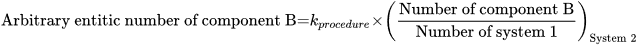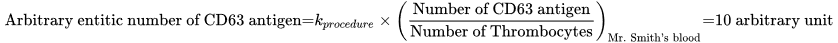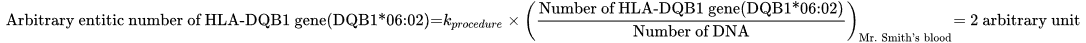Select Page

# Arbitrary entitic number (arb.entitic num.)

Arb.entitic num.

### Definition in words

1. Arbitrary entitic number of component B in system 1 (Arbitrary number of component B divided by number of system 1) (Ratio scale)
2. Arbitrary entitic number of component B in system 1, compared with arbitrary entitic number of component B in a reference system (Ordinal scale)

Note 1: This concept has two definitions.

Note 2: The reference system is defined by the laboratory.

### Definition by equation

1.### Result type

Ordinal scale
• Value set is ranked accoding to magnitude
• The value set and the limit of the value set are defined by the laboratory
• The value set can be either numerical values or words.Example 1Value set={none < some < high < very high}Example 2Value set={low < normal< high }

Example 3

Value set={0 < 1 < 2}

Example 4

Value set={negative < positive}

Example 5

Value set={+ < ++ < +++}

• Algebraic operations are not allowed (Ref. 2, 1.26)
• Possible comparison of values (a<b, a=b or a>b)
Ratio scale
• Value sets are positive numerical values
• Numerical values from 0-∞
• Algebraic operations are allowed
• Possible comparison of values (a=nb; a≠nb)

### Unit

International unit (IU) or procedure defined unit (p.d.u.) (non-SI units)

### Special rule(s) in the NPU syntax

“Procedure” is stated in the specification to the kind-of-property in the NPU definition.

“Procedure” indicates that the measurement procedure must be stated somewhere else than the NPU code and there may be difficulties in comparing values for the same NPU code.

If the ratio scale is attributed to the NPU definition, the unit can be non-SI unit.

If the unit is an International Unit (IU), an international certified reference material (e.g. WHO reference material) is specified in the specification to the kind-of-property.

If there exist no international certified reference material, the laboratory can specify the unit at the placeholder, procedure defined unit.

If ordinal scale is attributed, no unit is stated.

### Reference

1. Ferard G, Dybkaer R, Fuentes-Arderiu X. Compendium of Terminology and Nomenclature of Properties in Clinical Laboratory Sciences : Recommendations 2016. 1 ed: Royal Society of Chemistry; 2016. 182 p.
2. Mesures BIdPe. International Vocabulary of Metrology – Basic and General Concepts and Associated Terms VIM 3rd edition JCGM 200:2012 (JCGM 200:2008 with minor corrections). https://www.bipm.org: Bureau International des Poids et Mesures; 2008.

### Examples

Clinical Immunology and Blood Banking Example 1 (ordinal)
Generic abbreviated form NPU54860 Trcs(B)—Integrin beta-3; arb.entitic num.(proc.) = ?
Full form with a result NPU54860 Thrombocytes(Blood)—Integrin beta-3; arbitrary entitic number(procedure) = ++
Abbreviated form with a result NPU54860 Trcs(B)—Integrin beta-3; arb.entitic num.(proc.) = ++
Written expression The arbitrary number of Integrin beta-3 per thrombocyte in Mr Smith’s blood is [++], compared with arbitrary number of Integrin beta-3 per thrombocyte in a reference system. The measurement is performed according to a procedure defined by the given laboratory.
Notes
1. The reference system is defined by the laboratory.
2. “++” is a value according to a value set defined by the laboratory.
Example 2 (ratio)
Generic abbreviated form NPU28321 Trcs(B)—CD63 antigen; arb.entitic num.(proc.) = ? (p.d.u.)
Full form with a result NPU28321 Thrombocytes(Blood)—CD63 antigen; arbitrary entitic number(procedure) = 10 arbitrary unit
Abbreviated form with a result NPU28321 Trcts(B)—CD63 antigen; arb.entitic num.(proc.) = 10 arbitrary unit
Written expression The arbitrary number of CD63 antigen per thrombocyte in Mr Smith’s blood is  arbitrary unit. The measurement is performed according to a procedure defined by the given laboratory.
EquationNotes “Arbitrary unit” was chosen by a local laboratory as a proprietary measurement unit and not an accepted international unit.

Molecular Biology and Genetics

Example 3 (ordinal)
Generic abbreviated form NPU58663 DNA(B)—KIT gene(LRG_307t1:c.2446G>C); arb. entitic number(procedure) = ?
Full form with a result NPU58663 DNA(Blood)—KIT gene(LRG_307t1:c.2446G>C); arbitrary entitic number(procedure) = 1
Abbreviated form with a result

NPU58663 DNA(B)—KIT gene(LRG_307t1:c.2446G>C); arb. entitic number(procedure) = 1

Written expression The arbitrary number of KIT gene(LRG_307t1:c.2446G>C) per DNA in Mr Smith’s blood is , compared with arbitrary number of KIT gene(LRG_307t1:c.2446G>C) per DNA in a reference system. The measurement is performed according to a procedure defined by the given laboratory.
Notes
1. The reference system is defined by the laboratory.
2. “1” is a value according to a value set defined by the laboratory.
Example 4 (ratio)
Generic abbreviated form NPU53049 DNA(spec.)—HLA-DQB1 gene(DQB1*06:02); arb.entitic num.(proc.) = ?
Full form with a result NPU53049 DNA(spec.)—HLA-DQB1 gene(DQB1*06:02); arb.entitic num.(proc.) = 2 arbitrary unit
Abbreviated form with a result NPU53049 DNA(spec.)—HLA-DQB1 gene(DQB1*06:02); arb.entitic num.(proc.) = 2 arbitrary unit
Written expression The arbitrary number of CD63 antigen per thrombocyte in Mr Smith’s blood is  arbitrary unit. The measurement is performed according to a procedure defined by the given laboratory.
EquationNotes Arbitrary unit” was chosen by a local laboratory as a proprietary measurement unit and not an accepted international unit.
Reference NPU database, https://www.ifcc.org/ifcc-scientific-division/sd-committees/c-npu/npusearch/ and http://www.labterm.dk/default.aspx

• # Kind-of-property

• ## Kind-of-quantity

• Ordinal kind-of-quantity
• #### Arbitrary entitic number

• Unitary kind-of-quantity

### Reference

• Dybkaer R. Concept system on ‘quantity’: formation and terminology. Accredit Qual Assur. 2013;18(3):253-60.
• Dybkaer R. ISO terminological analysis of the VIM3 concepts ‘quantity’ and ‘kind-of-quantity’. Metrologia. 2010;47(3):127-34.
Date Term Note
1996-01-01 Arbitrary entitic number Term established.

QU50110